tensorflow 之循环神经网络

# RNN

RNN（Recurrent Neural Networks,循环神经网络）不仅会学习当前时刻的信息，也会依赖之前的序列信息。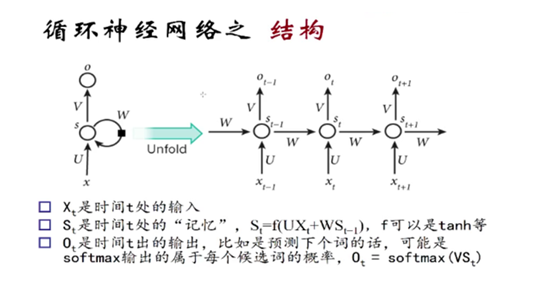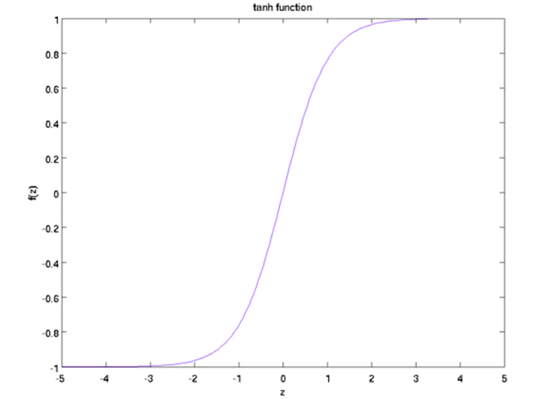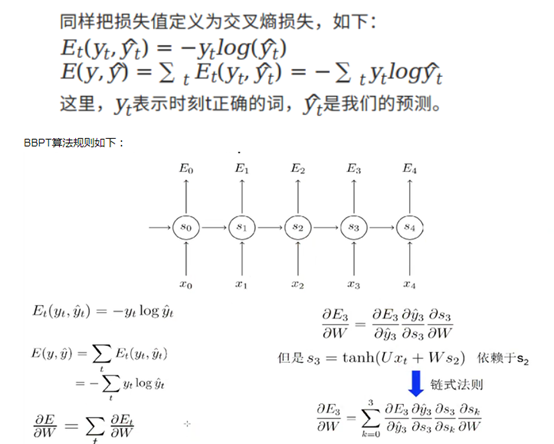RNN的激活函数tanh可以将所有值映射到-1至1之间，以及在利用梯度下降算法调优时利用链式法则，那么会造成很多个小于1的项连乘就很快的逼近零。

# LSTM

LSTM (Long Short Term Memory networks)的“门”结构可以截取“不该截取的信息”，结构如下：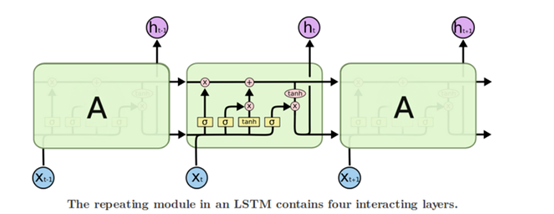LSTMs核心理念

LSTMs的关键点是细胞状态，就是穿过图中的水平线。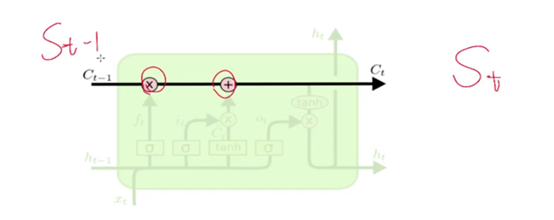LSTM有能力向单元状态中移除或添加信息，通过门结构来管理，包括“遗忘门”，“输出门”，“输入门”。通过门让信息选择性通过，来去除或增加信息到细胞状态. 模块中sigmoid层输出0到1之间的数字，描述了每个成分应该通过门限的程度。0表示“不让任何成分通过”，而1表示“让所有成分通过！”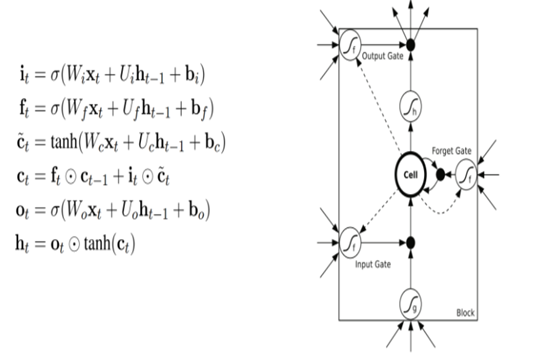LSTM循环神经网络分步说明: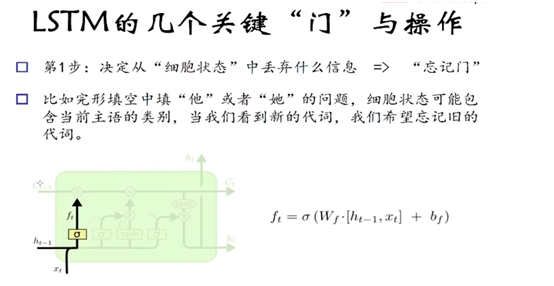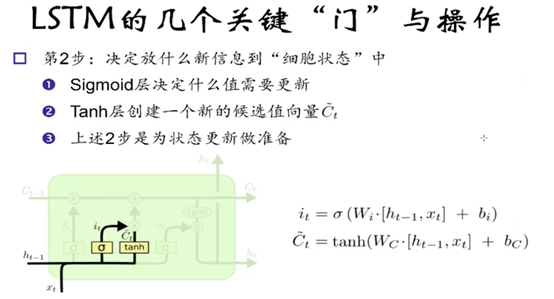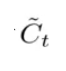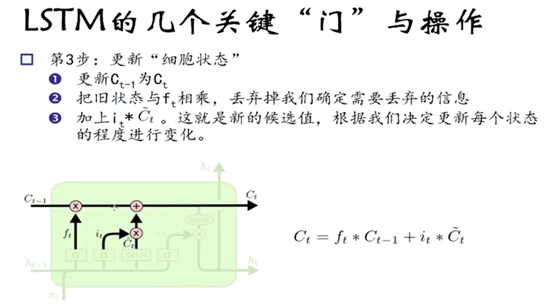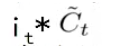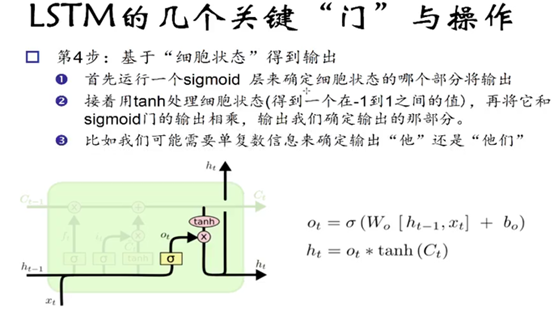Sigmoid函数的输出是不考虑先前时刻学到的信息的输出，tanh函数是对先前学到的信息的压缩处理，起到稳定数值的作用，两者的结合就是循环神经网络的学习思想。

# 代码演示

``````"""
demo 使用单层 LSTM网络 对 MNIST数据集分类

RNN网络应用领域：

lstm 长短期记忆网络
gru 闸门重复单元
"""

import tensorflow as tf
import numpy as np
# 导入 MINST 数据集
from tensorflow.examples.tutorials.mnist import input_data
mnist = input_data.read_data_sets("MNIST_data/", one_hot=True) # 在线下载 MNIST_data 数据集

n_input = 28 # MNIST data 输入 (img shape: 28*28)
n_steps = 28 # 序列个数
n_hidden = 128 # hidden layer num of features
n_classes = 10  # MNIST 列别 (0-9 ，一共10类)

tf.reset_default_graph()

# tf Graph input
x = tf.placeholder("float", [None, n_steps, n_input])
y = tf.placeholder("float", [None, n_classes])

x1 = tf.unstack(x, n_steps, 1)

#1 BasicLSTMCell  0.9453125
lstm_cell = tf.contrib.rnn.BasicLSTMCell(n_hidden, forget_bias=1.0)
outputs, states = tf.contrib.rnn.static_rnn(lstm_cell, x1, dtype=tf.float32)

#2 LSTMCell  0.9609375
# lstm_cell = tf.contrib.rnn.LSTMCell(n_hidden, forget_bias=1.0)
# outputs, states = tf.contrib.rnn.static_rnn(lstm_cell, x1, dtype=tf.float32)

#3 gru 0.9921875
# gru = tf.contrib.rnn.GRUCell(n_hidden)
# outputs = tf.contrib.rnn.static_rnn(gru, x1, dtype=tf.float32)

#4 创建动态RNN 0.9921875
# outputs,_  = tf.nn.dynamic_rnn(gru,x,dtype=tf.float32)
# outputs = tf.transpose(outputs, [1, 0, 2])

pred = tf.contrib.layers.fully_connected(outputs[-1],n_classes,activation_fn = None)

learning_rate = 0.001
training_iters = 100000
batch_size = 128
display_step = 10

# Define loss and optimizer
cost = tf.reduce_mean(tf.nn.softmax_cross_entropy_with_logits(logits=pred, labels=y))

# Evaluate model
correct_pred = tf.equal(tf.argmax(pred,1), tf.argmax(y,1))
accuracy = tf.reduce_mean(tf.cast(correct_pred, tf.float32))

# 启动session
with tf.Session() as sess:
sess.run(tf.global_variables_initializer())
step = 1
# Keep training until reach max iterations
while step * batch_size < training_iters:
batch_x, batch_y = mnist.train.next_batch(batch_size)
# Reshape data to get 28 seq of 28 elements
batch_x = batch_x.reshape((batch_size, n_steps, n_input))
# Run optimization op (backprop)
sess.run(optimizer, feed_dict={x: batch_x, y: batch_y})
if step % display_step == 0:
# 计算批次数据的准确率
acc = sess.run(accuracy, feed_dict={x: batch_x, y: batch_y})
# Calculate batch loss
loss = sess.run(cost, feed_dict={x: batch_x, y: batch_y})
print ("Iter " + str(step*batch_size) + ", Minibatch Loss= " + \
"{:.6f}".format(loss) + ", Training Accuracy= " + \
"{:.5f}".format(acc))
step += 1
print (" Finished!")

# 计算准确率 for 128 mnist test images
test_len = 128
test_data = mnist.test.images[:test_len].reshape((-1, n_steps, n_input))
test_label = mnist.test.labels[:test_len]
print ("Testing Accuracy:", \
sess.run(accuracy, feed_dict={x: test_data, y: test_label}))
``````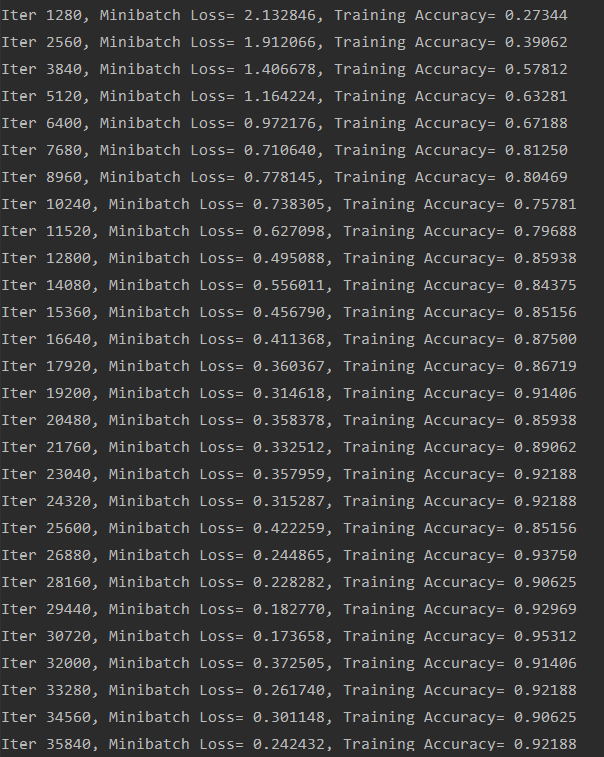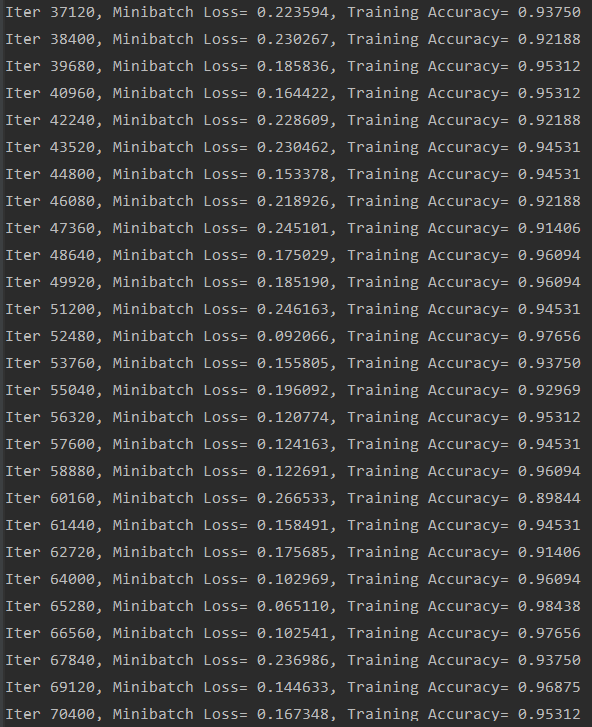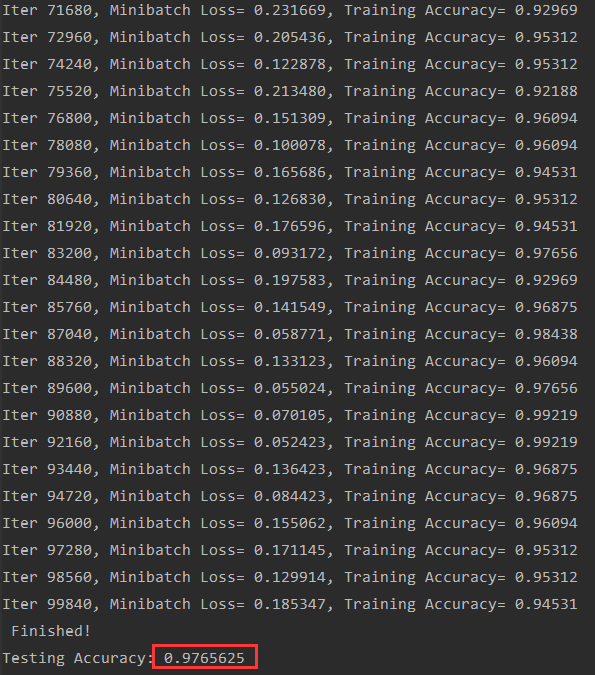blmius
1年前
MySQL:[Err] 1292 - Incorrect datetime value: ‘0000-00-00 00:00:00‘ for column ‘CREATE_TIME‘ at row 1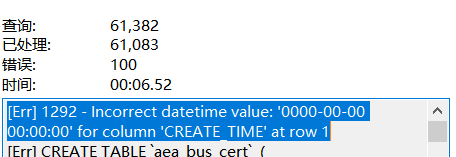Jacquelyn38
1年前
2020年前端实用代码段，为你的工作保驾护航Stella981
1年前
KVM调整cpu和内存Stella981
1年前
Python之time模块的时间戳、时间字符串格式化与转换
Python处理时间和时间戳的内置模块就有time，和datetime两个，本文先说time模块。关于时间戳的几个概念时间戳，根据1970年1月1日00:00:00开始按秒计算的偏移量。时间元组（struct_time），包含9个元素。 time.struct_time(tm_yWesley13
1年前
Java日期时间API系列36
十二时辰，古代劳动人民把一昼夜划分成十二个时段，每一个时段叫一个时辰。二十四小时和十二时辰对照表：时辰时间24时制子时深夜11：00凌晨01：0023：0001：00丑时上午01：00上午03：0001：0003：00寅时上午03：00上午0Wesley13
1年前
MySQL查询按照指定规则排序
1.按照指定(单个)字段排序selectfromtable_nameorderiddesc;2.按照指定(多个)字段排序selectfromtable_nameorderiddesc,statusdesc;3.按照指定字段和规则排序selecStella981
1年前Stella981
1年前
Angular material matWesley13
1年前
MySQL部分从库上面因为大量的临时表tmp_table造成慢查询helloworld_34035044
8个月前

​在我们用皕杰报表工具设计填报报表时，如何在新增行里自动增加id呢？能新增整数排序id吗？目前可以在新增行里自动增加id，但只能用uuid函数增加UUID编码，不能新增整数排序id。uuid函数说明：获取一个UUID，可以在填报表中用来创建数据ID语法：uuid()或uuid(sep)参数说明：sep布尔值，生成的uuid中是否包含分隔符''，缺省为Easter79
Lv1

2.8k

2

0